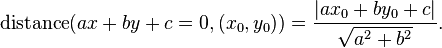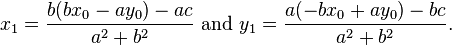## Distance from a point to a line calculator

 Line: X +  Y = Point: ,
 Distance :

The distance from a point to a line also called perpendicular distance is the shortest distance from a point to a line.

### Distance from a point to a line formula:

The equation ax + by + c = 0, the distance from the line to a point (x0,y0) isIf the point on this line which is closest to (x0,y0) has coordinates (x1,y1), then:For example, Line: x + 6y = 9, point (2,4), the distance from the line to the point is 2.7948.

Thinkcalculator.com provides you helpful and handy calculator resources.